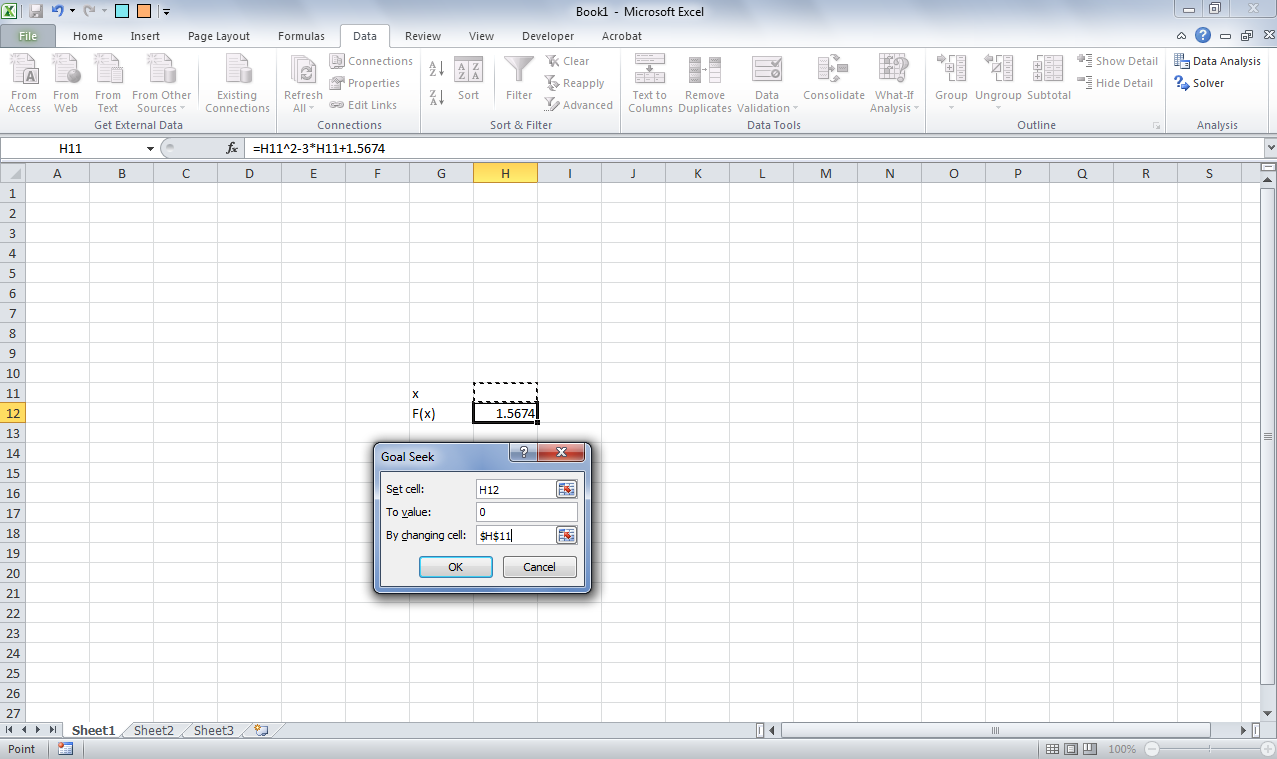### How to solve equations in Excel?

Many a times we encounter problems of solving equations within spreadsheet for engineering calculations. Suppose you want to solve n²-3n+1.5674 = 0, and want to find answer of the same in the middle of your engineering sum.

There are two options available in excel for the same.
1.       You can use goal seek. Follow following procedure for using goal seek.As shown in picture above you want x value for f(x) being 0.
a.       Go to Data -> What if analysis -> Goal seekb.      In set cell provide cell reference of F(x) (you want F(x) to be 0, right?)
c.       In to value type 0.
d.      In by changing cell provide cell reference of cell of x (you want value of x for which f(x) becomes 0.)
e.      Press ok and see the result.
f.        As we know it should give us two options for which f(x) becomes 0. But, goal seek provides least value for which f(x) becomes 0.

2. You can use solver option for the solution.